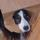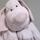## Social Question# What do you think of this math explanation?

Asked by LostInParadise (30680) October 25th, 2013

It is sometimes taught in high school that if you take the point (a,b) where a > 0 and b > 0 and rotate it 90 degrees along the circle with center at (0,0), you get the point (-b,a).

I was trying to think of a visual explanation and came up with this Form the rectangle having vertices (a,b), (a,0), (0,b) and (0,0). When you rotate the point, drag the rectangle along with it. The image of the rectangle vertices along the axes is (0,0), (-b,0) and (0,a). Therefore the coordinates of the image of the point must be (-b,a).

This relates to the fact that the slope of a line perpendicular to a line with slope m has slope -1/m. The slope of the line joining (a,b) to the origin is m = b/a and the slope of the perpendicular is -a/b = -1/m.

Observing members: 0Composing members: 0Math is evil.

ccrow (8097)“Great Answer” (0) Flag as…I am probably just talking to myself, seeing the general lack of interest, but I created a dynamic display here It will probably prompt you to ask if you want to see it. There is nothing malicious here. You may then have to do a screen refresh to see the image.

Rotate the yellow point above by rotating the point on the circular control on the bottom. This gives a better way of seeing what happens when the rotation is 90 degrees.

LostInParadise (30680)“Great Answer” (1) Flag as…I think the visual works quite well.

To me, the explanation is similar to understanding one could keep the point at the same place and just turn the axes by 90 degrees in the opposite direction..

I do have an issue, though, with your rotation itself. From my point of view you are rotating by minus 90 degrees. Your (positive) 90 degree rotation would result in: (a,b) > (b,-a) > (-a,-b) > (-b,a), right?
:-)

whitenoise (14152)“Great Answer” (2) Flag as…Thanks. I am glad to see someone is paying attention. II was going to originally do it in terms of rotating the axes, but I thought this way might be easier to understand.

Counterclockwise is considered positive for some odd reason.

LostInParadise (30680)“Great Answer” (0) Flag as…Interesting. It has been 20+ years. Now you say, I remember it had to do with the axes. A positive turn would increase the second coordinate if the first one was positive, or something like that.

whitenoise (14152)“Great Answer” (0) Flag as…